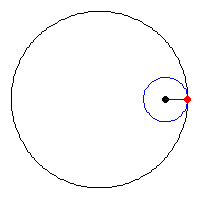# Astroid$\large \left(\dfrac{x}{a}\right)^{\frac{2}{3}} + \left(\dfrac{y}{a}\right)^{\frac{2}{3}} = 1$

The equation above shows an equation of an astroid with positive constant $a$, find the area enclosed by the astroid in terms of $a$.

×